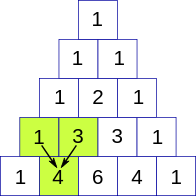# Pascal's Triangle

Posted: 11 Jan, 2021
Difficulty: Easy

## PROBLEM STATEMENT

#### A Pascal's triangle is a triangular array constructed by summing adjacent elements in preceding rows. Pascal's triangle contains the values of the binomial coefficient. For example in the figure below.#### For example, given integer N= 4 then you have to print.

``````1
1 1
1 2 1
1 3 3 1

Here for the third row, you will see that the second element is the summation of the above two-row elements i.e. 2=1+1, and similarly for row three 3 = 1+2 and 3 = 1+2.
``````
##### Input format :
``````The first line of input contains an integer ‘T’ denoting the number of test cases.
The first line of each test case contains a single integer N denoting the row till which you have to print the pascal’s triangle.
``````
##### Output format :
``````For each test case, return the 2-D array/list containing the pascal’s triangle till the row N.
``````
##### Note:
``````You do not need to print anything; it has already been taken care of. Just implement the given function.
``````
##### Constraints:
``````1 <= T <= 40
1 <= N <= 50

Time Limit: 1 sec
``````Approach 1

The idea is to use recursion to get the value of the coefficients we will create a helper function CALPASCAL which will take row and entry as its input parameters and we call this function in the main function of PRINTPASCAL.

• Inside the function CALPASCAL if the row is 0 or the entry is equal to row then we will just return 1.
• Else we will return the sum of adjacent previous row value that is CALPASCAL(I-1,J-1) + CALPASCAL(I-1,J).
• Now for the main function to store the pascal’s triangle PRINTPASCAL inside this declare a 2-D arrayList TRIANGLE  and run loop from row I=0 to I<N.
• Inside this loop run a loop from entry J=0 till entry J=R.
• Inside this loop calculate the answer for each entry by calling the CALPASCAL function and add it to the arraylist.
• Finally return the arraylist.